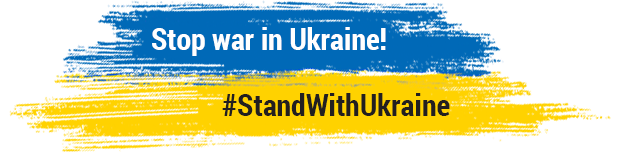foundations of mathematics, philosophy of mathematics, logic, model, modeling, modelling, mathematical, online, web, book, Internet, tutorial, textbook, foundations, mathematics, teaching, learning, study, mathematical logic, student, Podnieks, Karlis, philosophy, free, download

1935: Ludwig Wittgenstein visiting USSR

Visiting Gödel in Vienna, July 2014: Richard Zach determined the exact location of Cafe Reichsrat!

Mathematical Challenge (powers of 2, exponentiation, etc.)

Gödel's Theorem in 15 Minutes

Favorite Quotes (Grothendieck, Feynman, von Neumann, Zeilberger, Bohr, von Liebig, Einstein, Putnam, Turing, Friedman, Kolmogorov, ...)

## Introduction toMathematical Logic

Hyper-textbook for students
by Vilnis Detlovs and Karlis Podnieks
University of Latvia

Vilnis Detlovs. Memorial Page

## What is Mathematics:Gödel's Theorem and Around

Hyper-textbook for students
by Karlis Podnieks
Russian version available

## What is Mathematics?

Four provably equivalent definitions of mathematics:

Mathematics is the part of science you could continue to do if you woke up tomorrow and discovered the universe was gone.
This elegant definition was put on the web by Dave Rusin. Who might be the author?

It seems that the human mind has first to construct forms independently, before we can find them in things. Kepler’s marvelous achievement is a particularly fine example of the truth that knowledge cannot spring from experience alone, but only from the comparison of the inventions of the intellect with observed fact.
Albert Einstein
über Kepler. Frankfurter Zeitung, 9. November 1930
(English translation by Sonja Bargmann published in:
A. Einstein. Ideas and Opinions.
Crown Publishers, New York, 1954)

In mathematics you don't understand things. You just get used to them.
John von Neumann, see Quotations by John von Neumann).

Mathematicians are mad tailors: they are making "all the possible clothes" hoping to make also something suitable for dressing...
Stanislaw Lem, "Summa Technologiae" (sorry - my own English translation, the initial version of this aphorism may be due to David van Dantzig, see Quotations by David van Dantzig)

What is Mathematics? (My Main Theses)

I define mathematical theories as stable self-contained (autonomous?) systems of reasoning, and formal theories - as mathematical models of such systems. Working with stable self-contained models mathematicians have developed their ability to draw a maximum of conclusions from a minimum of premises. This is why mathematical modeling is so efficient (my solution to the problem of "The Incomprehensible Effectiveness of Mathematics in the Natural Sciences" (as put by Eugene Wigner).

For me, Goedel's results are the crucial evidence that stable self-contained systems of reasoning cannot be perfect (just because they are stable and self-contained). Such systems are either non-universal (i.e. they cannot express the notion of natural numbers: 0, 1, 2, 3, 4, ...), or they are universal, yet then they run inevitably either into contradictions, or into unsolvable problems.

For humans, Platonist thinking is the best way of working with imagined structures. (Another version of this thesis was proposed in 1991 by Keith Devlin on p. 67 of his Logic and Information.) Thus, a correct philosophical position of a mathematician should be: a) Platonism - on working days - when I'm doing mathematics (otherwise, my "doing" will be inefficient), b) Formalism - on weekends - when I'm thinking "about" mathematics (otherwise, I will end up in mysticism). (The initial version of this aphorism was proposed in 1979 by Reuben Hersh (picture) on p. 32 of his Some proposals for reviving the philosophy of mathematics.)

Next step

The idea that stable self-contained system of basic principles is the distinctive feature of mathematical theories, can be regarded only as the first step in discovering the nature of mathematics. Without the next step, we would end up by representing mathematics as an unordered heap of mathematical theories!

In fact, mathematics is a complicated system of interrelated theories each representing some significant mathematical structure (natural numbers, real numbers, sets, groups, fields, algebras, all kinds of spaces, graphs, categories, computability, all kinds of logic, etc.).

Thus, we should think of mathematics as a "two-dimensional" activity. Sergei Yu. Maslov could have put it as follows: most of a mathematician's working time is spent along the first dimension (working in a fixed mathematical theory, on a fixed mathematical structure), but, sometimes, he/she needs also moving along the second dimension (changing his/her theories/structures or, inventing new ones).

Do we need more than this, to understand the nature of mathematics?

John Keats (1795 – 1821), thanks to Anna Akhmatova.

"I have left no immortal work behind me — nothing to make my friends proud of my memory - but I have lov'd the principle of beauty in all things, and if I had had time I would have made myself remember'd".

Isabella; or, The Pot of Basil

...

XXXIX.

“I am a shadow now, alas! alas!
“Upon the skirts of human-nature dwelling
“Alone: I chant alone the holy mass,
“While little sounds of life are round me knelling,
“And glossy bees at noon do fieldward pass,
“And many a chapel bell the hour is telling,
“Paining me through: those sounds grow strange to me,
“And thou art distant in Humanity.

...

foundations of mathematics, philosophy of mathematics, logic, model, modeling, modelling, mathematical, online, web, book, Internet, tutorial, textbook, foundations, mathematics, teaching, learning, study, mathematical logic, student, Podnieks, Karlis, philosophy, free, download